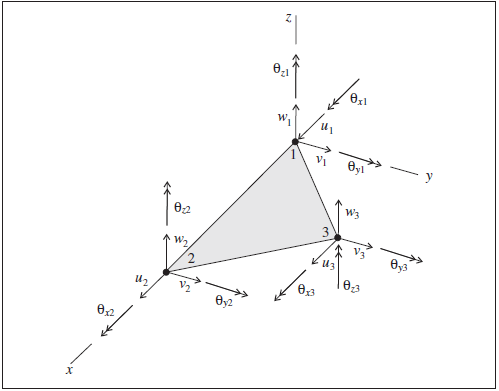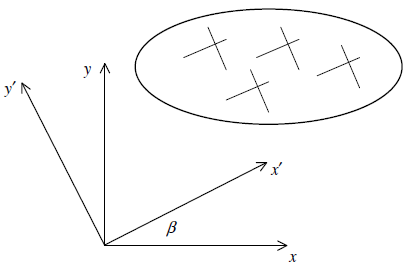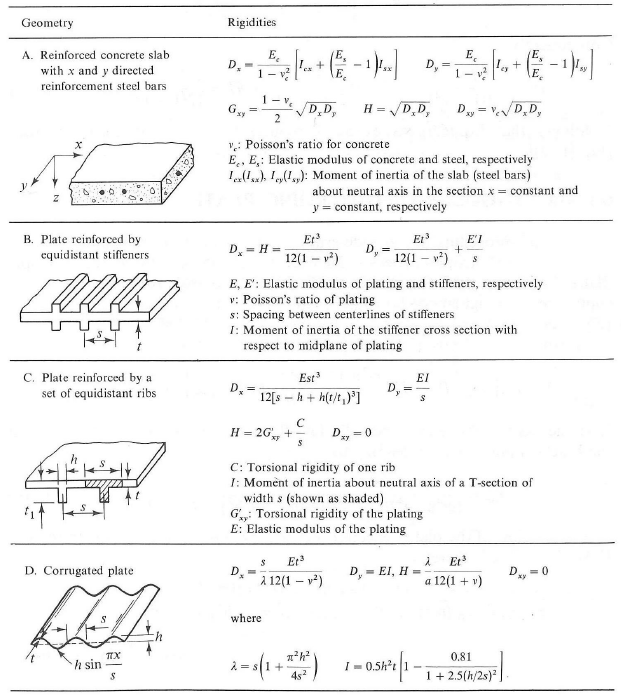# Shell-Type Structural Elements

The shell-type structural elements include shell elements, geogrid elements, and liner elements. The mechanical behavior of these elements can be divided into the structural response of the shell material itself, and the way the element interacts with the grid. The structural response of the shell material is common to all shell-type elements, and is described in this section. Specific behaviors that differ for each element type are described in the section for that type.

## Mechanical Behavior

Each shell-type structural element (shell, geogrid, or liner) is defined by its geometric and material properties. A shell-type element is assumed to be a triangle of uniform thickness lying between three nodal points. An arbitrarily curved structural shell can be modeled as a faceted surface composed of a collection of shell-type elements. Each shell-type element behaves as an isotropic or anisotropic, linearly elastic material with no failure limit; however, one can introduce a plastic-hinge line (across which a discontinuity in rotation may develop) along the edges between shell-type elements, using the same double-node procedure as applied to beams (see Plastic Hinge Formation in a Shell Structure). Each shell-type element provides a different means of interacting with the grid (see Shell Structural Elements, Geogrid Structural Elements, and Liner Structural Elements). The structural response of the shell is controlled by the finite element assigned to the element. There are five finite elements available: 2 membrane elements, 1 plate-bending element and 2 shell elements. The general properties of these finite elements are described in Shell Finite Elements. Because these are all thin-shell finite elements, shell-type elements are suitable for modeling thin-shell structures in which the displacements caused by transverse-shearing deformations can be neglected. Thick-shell structures should be modeled with zones.

Each shell-type element has its own local coordinate system shown in Figure 1. This system is used to specify applied pressure loading. A separate material coordinate system is used to specify orthotropic material properties, and a surface coordinate system (providing a continuous description of the shell mid-surface spanning adjacent shell-type elements) is used to recover stresses — see Stresses in Shells. The shell-type element coordinate system is defined by the locations of its three nodal points, labeled 1, 2 and 3 in Figure 1. The shell-type element coordinate system is defined such that

1. the shell-type element lies in the $$xy$$-plane,
2. the $$x$$-axis is directed from node-1 to node-2, and
3. the $$z$$-axis is normal to the element plane and positive on the “outside” of the shell surface. (The two sides of each shell-type element are designated as outside and inside.)

The shell-type element coordinate system cannot be modified.Figure 1: Shell-type element coordinate system and 18 degrees of freedom available to the shell finite elements

## Response Quantities

Stress quantities (which include stress resultants and stresses acting in the shell) can be recovered for all shell-type elements. The stress resultants are expressed in a surface coordinate system that provides a continuous description of the shell mid-surface spanning adjacent shell-type elements. The stresses are expressed in the global coordinate system. Stress quantities can only be recovered after a consistent surface coordinate system has been established (see Stress Recover Procedure). The stress quantities can be accessed via FISH, and

1. printed with the structure shell list stress or structure shell list stress-principal commands, or the equivalent for geogrid or liner elements.,
2. monitored with the structure shell history command (or equivalent for geogrid or liner elements), and
3. plotted with the Shell, Geogrid, or Liner plot items.

Note that the stresses and stress-resultants calculated with the structure shell recover (for example) command are the values stored in the element and available to FISH. By default, the Shell-type plot items calculate stress resultant quantities independently. It is possible to instruct the plot items to display the values calculated with the structure shell recover command, in which case they will not be update until the next command is issued.

## Properties

Each shell-type element possesses 4 properties:

1. density, mass density, $$\rho$$ (optional — needed if dynamic mode or gravity is active) [M/L3]

2. Isotropic:

isotropic, isotropic material properties: $$E$$ and $$\nu$$ where $$E$$ is Young’s modulus [F/L2] and $$\nu$$ is Poisson’s ratio.

Orthotropic:

orthotropic-membrane, orthotropic material properties, {$$c^{\prime}_{11}, c^{\prime}_{12}, c^{\prime}_{22}, c^{\prime}_{33}$$} $$[F/L^2]$$ which defines the membrane material stiffness matrix $$[{{\bf E}_m}^{\prime}]$$ in the material coordinate system.

orthotropic-bending, orthotropic material properties, {$$c^{\prime}_{11}, c^{\prime}_{12}, c^{\prime}_{22}, c^{\prime}_{33}$$} $$[F/L^2]$$ which defines the bending material stiffness matrix $$[{{\bf E}_b}^{\prime}]$$ in the material coordinate system.

Anisotropic:

anisotropic-membrane, anisotropic membrane material properties, {$$c^{\prime}_{11}, c^{\prime}_{12}, c^{\prime}_{13}, c^{\prime}_{22}, c^{\prime}_{23}, c^{\prime}_{33}$$} $$[F/L^2]$$, which defines the membrane material-stiffness matrix $$[Eʹ_m]$$ in the material coordinate system.

anisotropic-bending, anisotropic bending material properties, {$$c^{\prime}_{11}, c^{\prime}_{12}, c^{\prime}_{13}, c^{\prime}_{22}, c^{\prime}_{23}, c^{\prime}_{33}$$} $$[F/L^2]$$, which defines the membrane bending-stiffness matrix $$[Eʹ_b]$$ in the material coordinate system.

3. thermal-expansion, thermal-expansion coefficient, $$\alpha_t$$ [1/T]

4. thickness, thickness, $$t$$ [L]

The material constitutive behavior may be isotropic, orthotropic or anisotropic; thus, one of property (2a) through (2g) must be specified. It is assumed that the material properties are homogeneous over the shell-type element (i.e., they do not vary with position) and that the shell thickness is constant. A description of the material properties follows.

The shell-type elements model general shell behavior as a superposition of membrane and bending actions via the five 3-noded triangular finite elements described in Shell Finite Elements. The material properties of the finite elements that model membrane and bending actions are described by the material-rigidity matrices

(1)$\begin{split}\begin{split} [{\bf D}_m] &= \int_{-{t/2}}^{+{t/2}} [{\bf E}_m]dz = t[{\bf E}_m] \\ [{\bf D}_b] &= \int_{-{t/2}}^{+{t/2}} [{\bf E}_b]z^2dz = {{t^3} \over {12}} [{\bf E}_b] \end{split}\end{split}$

respectively, where $$t$$ is the shell thickness and $$[{\bf E}_m]$$ and $$[{\bf E}_b]$$ are material-stiffness matrices that relate stresses to strains via the constitutive relations

(2)\begin{align}\begin{aligned}\begin{split}\begin{Bmatrix} \sigma_m \end{Bmatrix} = {\begin{Bmatrix} \sigma_x \\ \sigma_y \\ \tau_{xy} \end{Bmatrix}}_m = \begin{bmatrix} {\pmb E}_m \end{bmatrix} \begin{Bmatrix} {\pmb \varepsilon} \end{Bmatrix} = \begin{bmatrix} c^m_{11} & c^m_{12} & c^m_{13} \\ & c^m_{22} & c^m_{23} \\ sym. & & c^m_{33} \end{bmatrix} \begin{Bmatrix} \varepsilon_x \\ \varepsilon_y \\ \gamma_{xy} \end{Bmatrix}\end{split}\\\begin{split}\begin{Bmatrix} \sigma_b \end{Bmatrix} = {\begin{Bmatrix} \sigma_x \\ \sigma_y \\ \tau_{xy} \end{Bmatrix}}_b = \begin{bmatrix} {\pmb E}_b \end{bmatrix} \begin{Bmatrix} {\pmb \varepsilon} \end{Bmatrix} = \begin{bmatrix} c^b_{11} & c^b_{12} & c^b_{13} \\ & c^b_{22} & c^b_{23} \\ sym. & & c^b_{33} \end{bmatrix} \begin{Bmatrix} \varepsilon_x \\ \varepsilon_y \\ \gamma_{xy} \end{Bmatrix}\end{split}\end{aligned}\end{align}

The material-rigidity matrices are used to form the finite element stiffness matrices ($$[{\bf D}_m]$$ is used by the CST and CST hybrid finite elements, and $$[{\bf D}_b]$$ is used by the DKT finite element) and to recover stress resultants. The stresses in (2) are obtained from the stress resultants by

$\begin{split}\begin{split} \begin{Bmatrix} \sigma_m \end{Bmatrix} &= \frac{1}{t} \begin{Bmatrix} N_x \\ N_y \\ N_{xy} \end{Bmatrix} \\ \begin{Bmatrix} \sigma_b \end{Bmatrix} &= \frac{12}{t^3} \begin{Bmatrix} M_x \\ M_y \\ M_{xy} \end{Bmatrix} z \end{split}\end{split}$

In the following discussion, we use $$[{\bf E}]$$ when referring to relations that apply to both $$[{\bf E}_b]$$ and $$[{\bf E}_m]$$. For isotropic material properties, only $$E$$, $$v$$ and $$t$$ must be specified. For orthotropic and anisotropic material properties, the material directions and $$t$$ must be specified. For most cases, $$[{\bf E}_m] = [{\bf E}_b] = [{\bf E]}$$; however, when modeling equivalent or transformed orthotropic shells (with elastic properties equal to the average properties of components of the original shell) and controlling the membrane and bending rigidities independently, it is necessary to set $$[{\bf E}_m] \not= [{\bf E}_b]$$.

Isotropic Material Properties

For the case of an isotropic shell, $$[{\bf E}_m] = [{\bf E}_b] = [{\bf E}]$$, and the six constants of $$[{\bf E}]$$ are related to the two elastic constants of Young’s modulus, $$E$$, and Poisson’s ratio, $$\nu$$, by

$\begin{split}\begin{split} c_{11} &= c_{22} = {E \over 1 - \nu^2} \\ c_{33} &= {E \over 2(1 + \nu)} \\ c_{12} &= \nu\left({E \over 1 - \nu^2}\right) \\ c_{13} &= c_{23} = 0 \end{split}\end{split}$

The $$c_{ij}$$ are invariant constants and retain the same values in any orthogonal coordinate system.

Orthotropic and Anisotropic Material Properties

Under the assumptions of linear elasticity, the general constitutive matrix of material stiffness coefficients is symmetric and can be expressed in terms of 21 independent elastic constants. An orthotropic material has three preferred directions of elastic symmetry, and its material-stiffness matrix can be expressed in terms of 9 independent elastic constants:

(3)$\begin{split}\begin{Bmatrix} \sigma_{x^{\prime}} \\ \sigma_{y^{\prime}} \\ \sigma_{z^{\prime}} \\ \tau_{x^{\prime}y^{\prime}} \\ \tau_{x^{\prime}z^{\prime}} \\ \tau_{y^{\prime}z^{\prime}} \end{Bmatrix} = \begin{bmatrix} C^{\prime}_{11} & C^{\prime}_{12} & C^{\prime}_{13} & 0 & 0 & 0 \\ & C^{\prime}_{22} & C^{\prime}_{23} & 0 & 0 & 0 \\ & & C^{\prime}_{33} & 0 & 0 & 0 \\ & & & C^{\prime}_{44} & 0 & 0 \\ & sym. & & & C^{\prime}_{55} & 0 \\ & & & & 0 & C^{\prime}_{66} \end{bmatrix} \begin{Bmatrix} \varepsilon_{x^{\prime}} \\ \varepsilon_{y^{\prime}} \\ \varepsilon_{z^{\prime}} \\ \gamma_{x^{\prime}y^{\prime}} \\ \gamma_{x^{\prime}z^{\prime}} \\ \gamma_{y^{\prime}z^{\prime}} \end{Bmatrix}\end{split}$

in which $$x^{\prime} y^{\prime} z^{\prime}$$ are the principal directions of orthotropy. This relation describes a three-dimensional orthotropic continuum. The relation can be restricted to describe an orthotropic shell by enforcing the Kirchhoff thin-plate conditions of plane stress ($$\sigma_{z^{\prime}} = 0$$) and no transverse-shear strain ($$\gamma_{x^{\prime}z^{\prime}} = \gamma_{y^{\prime}z^{\prime}} = 0$$), so that the material-stiffness matrix can be expressed in terms of 4 independent elastic constants:

(4)$\begin{split}\begin{Bmatrix} \sigma^{\prime} \end{Bmatrix} = \begin{Bmatrix} \sigma_x^{\prime} \\ \sigma_y^{\prime} \\ \tau_{x^{\prime}y^{\prime}} \end{Bmatrix} = \begin{bmatrix} {\pmb E^{\prime}} \end{bmatrix} \begin{Bmatrix} {\pmb \varepsilon^{\prime}} \end{Bmatrix} = \begin{bmatrix} c^{\prime}_{11} & c^{\prime}_{12} & 0 \\ & c^{\prime}_{22} & 0 \\ sym. & & c^{\prime}_{33} \end{bmatrix} \begin{Bmatrix} \varepsilon_{x^{\prime}} \\ \varepsilon_{y^{\prime}} \\ \gamma_{x^{\prime}y^{\prime}} \end{Bmatrix} \rm {(orthotropic\ shell)}\end{split}$

in which the shell mid-surface lies in the $$x^{\prime} y^{\prime}$$-plane. The material-stiffness matrix expressed in the principal directions (denoted here by $$[{\bf E^{\prime}}]$$) has four independent constants that define an orthotropic material.

The material-stiffness matrix of an anisotropic shell can be expressed in terms of 6 independent elastic constants:

(5)$\begin{split}\begin{Bmatrix} \sigma^{\prime} \end{Bmatrix} = \begin{Bmatrix} \sigma_x^{\prime} \\ \sigma_y^{\prime} \\ \tau_{x^{\prime}y^{\prime}} \end{Bmatrix} = \begin{bmatrix} {\pmb E^{\prime}} \end{bmatrix} \begin{Bmatrix} {\pmb \varepsilon^{\prime}} \end{Bmatrix} = \begin{bmatrix} c^{\prime}_{11} & c^{\prime}_{12} & c^{\prime}_{13} \\ & c^{\prime}_{22} & c^{\prime}_{23} \\ sym. & & c^{\prime}_{33} \end{bmatrix} \begin{Bmatrix} \varepsilon_{x^{\prime}} \\ \varepsilon_{y^{\prime}} \\ \gamma_{x^{\prime}y^{\prime}} \end{Bmatrix} \rm {(anisotropic\ shell)}\end{split}$

in which the shell mid-surface lies in the $$x^{\prime} y^{\prime}$$-plane. The material-stiffness matrix expressed in the material directions (denoted here by $$[{\bf E^{\prime}}]$$) has six independent constants that define an anisotropic shell.

Consider a shell-type element with its local coordinate system, $$xyz$$, rotated with respect to the $$x^{\prime}$$-axis by an angle, $$\beta$$, as shown in Figure 2. The strain-transformation matrix, $$\begin{bmatrix}{\bf T_{\varepsilon}}\end{bmatrix}$$, relates the strains in the two systems via

(6)$\begin{Bmatrix} {\pmb \varepsilon^{\prime}} \end{Bmatrix} = \begin{bmatrix} {\bf T_{\bf \varepsilon}} \end{bmatrix} \begin{Bmatrix} {\pmb \varepsilon} \end{Bmatrix}$

$$\begin{bmatrix}{\bf T_{\varepsilon}}\end{bmatrix}$$ can be expressed in terms of $$\beta$$ as

$\begin{split}\begin{bmatrix} {\bf T_{\varepsilon}} \end{bmatrix} = \begin{bmatrix} c^2 & s^2 & cs \\ s^2 & c^2 & -cs \\ -2cs & 2cs & c^2 - s^2 \end{bmatrix}, \begin{matrix} c = {\rm cos} \beta \\ s = {\rm sin} \beta \end{matrix}\end{split}$

The stresses in the two systems are related via

$\begin{Bmatrix} {\bf \sigma} \end{Bmatrix} = \begin{bmatrix} {\bf T_{\varepsilon}} \end{bmatrix}^T \begin{Bmatrix} {\bf \sigma^{\prime}} \end{Bmatrix}$

Substituting (4) or (5) and (6) into the preceding yields

$\begin{Bmatrix} {\bf \sigma} \end{Bmatrix} = \begin{bmatrix} {\bf T_{\varepsilon}} \end{bmatrix}^T \begin{bmatrix} {\bf E^{\prime}} \end{bmatrix} \begin{bmatrix} {\bf T_{\varepsilon}} \end{bmatrix} \begin{Bmatrix} {\bf \varepsilon} \end{Bmatrix}$

in which

(7)$\begin{bmatrix} {\bf E} \end{bmatrix} = \begin{bmatrix} {\bf T_{\varepsilon}} \end{bmatrix}^T \begin{bmatrix} {\bf E^{\prime}} \end{bmatrix} \begin{bmatrix} {\bf T_{\varepsilon}} \end{bmatrix}$

Thus we have, from (7) and (1), the expression

$\begin{bmatrix} {\bf D}_m \end{bmatrix} = t \begin{bmatrix} {\bf T_{\varepsilon}} \end{bmatrix}^T \begin{bmatrix} {\bf E^{\prime}_m} \end{bmatrix} \begin{bmatrix} {\bf T_{\varepsilon}} \end{bmatrix}$

and

$\begin{bmatrix} {\bf D}_b \end{bmatrix} = \frac{t^3}{12} \begin{bmatrix} {\bf T_{\varepsilon}} \end{bmatrix}^T \begin{bmatrix} {\bf E^{\prime}_b} \end{bmatrix} \begin{bmatrix} {\bf T_{\varepsilon}} \end{bmatrix}$

for the material-rigidity matrices of a shell in which the material directions are not aligned with the $$x$$ and $$y$$ axes. For an orthotropic shell, $$[{\bf E^{\prime}}]$$ has two zero terms, but these terms will not, in general, be zero for $$[{\bf E}]$$ when the principal directions of orthotropy are not aligned with the $$x$$ and $$y$$ axes.Figure 2: Shell-type element coordinate system $$xyz$$ and material coordinate system $$x^{\prime}y^{\prime}z^{\prime}$$

Determination of Orthotropic Material Properties

The material-stiffness matrix $$[{{\bf E}_m}^{\prime}]$$ and $$[{{\bf E}_b}^{\prime}]$$ can be expressed in terms of the elastic constants in (3) via

$\begin{split}\begin{split} c^{\prime}_{11} &= C^{\prime}_{11} - {{C^{\prime}_{13} C^{\prime}_{13}} \over {C^{\prime}_{33}}} \\ c^{\prime}_{22} &= C^{\prime}_{22} - {{C^{\prime}_{23} C^{\prime}_{23}} \over {C^{\prime}_{33}}} \\ c^{\prime}_{33} &= C^{\prime}_{44} \\ c^{\prime}_{12} &= C^{\prime}_{12} - {{C^{\prime}_{13} C^{\prime}_{23}} \over {C^{\prime}_{33}}} \end{split}\end{split}$

They can also be expressed in terms of the effective Poisson’s ratios ($$\nu_{x^{\prime}}$$ and $$\nu_{y^{\prime}}$$), effective moduli ($$E^{\prime}_{x^{\prime}}$$ and $$E^{\prime}_{y^{\prime}}$$) and shear modulus ($$G$$) for orthotropic plates (Ugural 1981, p. 141):

(8)$\begin{split}\begin{split} c^{\prime}_{11} &= {{E^{\prime}_{x^{\prime}}} \over {1 - \nu_{x^{\prime}} \nu_{y^{\prime}}}} \\ c^{\prime}_{22} &= {{E^{\prime}_{y^{\prime}}} \over {1 - \nu_{x^{\prime}} \nu_{y^{\prime}}}} \\ c^{\prime}_{33} &= G \\ c^{\prime}_{12} &= {{E^{\prime}_{x^{\prime}} \nu_{y{\prime}}} \over {1 - \nu_{x^{\prime}} \nu_{y^{\prime}}}} = {{E^{\prime}_{y^{\prime}} \nu_{x{\prime}}} \over {1 - \nu_{x^{\prime}} \nu_{y^{\prime}}}} \end{split}\end{split}$

Ugural states that the orthotropic plate moduli and Poisson’s ratios are obtained by tension and shear tests, as in the case of isotropic materials.

When it is not possible to determine the material stiffnesses experimentally, an equivalent or transformed orthotropic plate (with elastic properties equal to the average properties of components of the original plate), for which the membrane stiffnesses are approximated with the relations in (8), and the bending stiffnesses are approximated with the following rigidities, can be used.

$\begin{split}\begin{split} {c^b_{11}}^\prime &= {12 \over t^3} D_{x^{\prime}} \\ {c^b_{22}}^\prime &= {12 \over t^3} D_{y^{\prime}} \\ {c^b_{33}}^\prime &= {12 \over t^3} G_{x^{\prime}y^{\prime}} \\ {c^b_{12}}^\prime &= {12 \over t^3} D_{x^{\prime}y^{\prime}} \end{split}\end{split}$

$$D_{x^{\prime}}$$, $$D_{y^{\prime}}$$, $$D_{x^{\prime}y^{\prime}}$$ and $$G_{x^{\prime}y^{\prime}}$$ represent the flexural rigidities and the torsional rigidity of an orthotropic plate, respectively. Rigidities for some commonly encountered cases are given in Figure 3. These cases include a reinforced concrete slab with orthogonal steel bars, a plate reinforced by equidistant stiffeners, a plate reinforced by a set of equidistant ribs, and a corrugated plate.Figure 3: Rigidities of various orthotropic plates (from Ugural 1981, Table 6.1, where $$H = 2G_{xy} + D_{xy}$$)*

Note

*Used with the permission of McGraw-Hill Publishing Company.